Waveguide Loss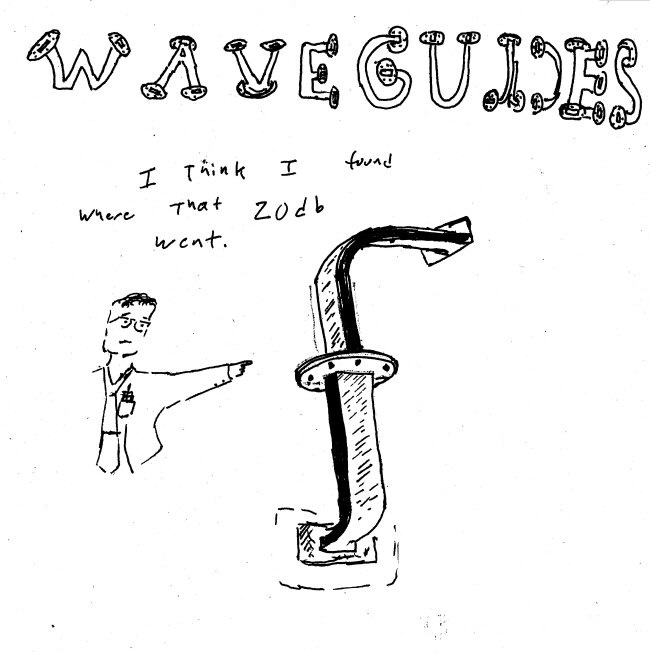This picture of crossed waveguides came from JB. It depicts a sorry situation that happens often in a microwave lab, especially in (but not limited to) setups by newer engineers. The flanges on many waveguides will allow you to connect them in a "cross-guided" fashion, resulting in a mysterious higher-than-expected loss (usually around 20 dB). The resulting horrendous VSWR plays havoc as well. If you click the picture you'll access a larger image, suitable for framing, we recommend you display it over the waveguide cabinet in your lab. Thanks, JB! Nice pocket protector!

Loss due to metal

The ideal metal loss for rectangular waveguide boils down to some neat closed-form equations. For this calculation we used Pozar's book, which covers the subject.

The first two things to calculate are the RF sheet resistance (function of the waveguide material's conductivity, permeability, and frequency).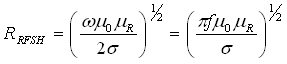Next we need to calculate the imaginary part of the propagation constant, beta. We're only going to consider the dominant TE10 mode. First we consider the cut-off wavenumber: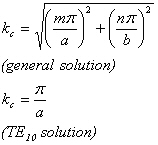Now we look at the wavenumber within the waveguide fill material (which is usually air):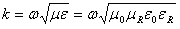Then the phase constant is: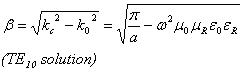Need to post the loss equations...

The loss goes to infinity at the lower cutoff frequency. Here's a plot of the loss of WR-90 X-band waveguide. Note that it blows up at the lower cutoff frequency of 6.557 GHz. In practice, the generally accepted frequency band limits for rectangular waveguide are between 125% and 189% of the lower cutoff frequency, in this case 8.2 to 12.4 GHz.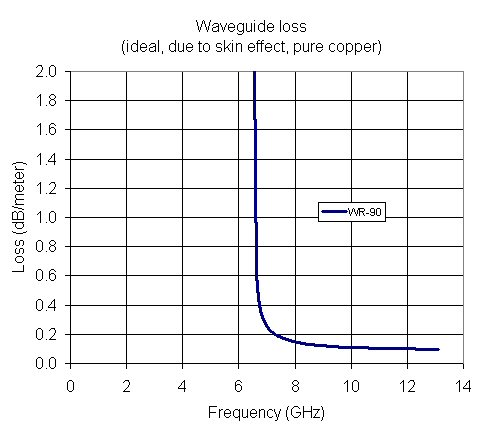While we are waiting for equations to develop, here's a plot of eight different waveguide sizes, using the conductivity of pure copper: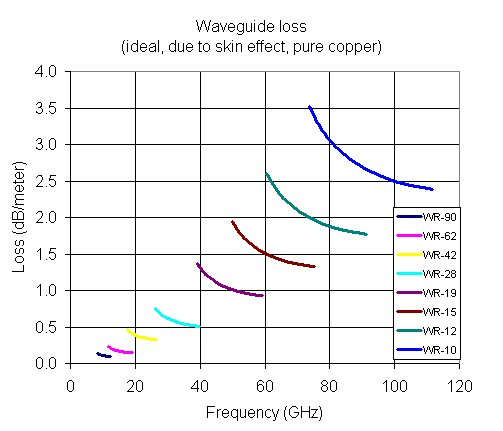This is one of the coolest plots in microwave engineering, waveguide vendors have been known to make it into a poster which hangs in hundreds of laboratories around the world. We did this one in Excel, if anyone wants a copy of the spreadsheet, just click here.  We've updated the spreadsheet for January 2015!

Because it's hard to read exact numbers from the chart, here are the losses at selected mid-band frequencies:

WR-90: 0.108 dB/m (10 GHz),
WR-62: 0.168 dB/m (15 GHz)
WF-42: 0.370 dB/m (21 GHz)
WR-28: 0.576 dB/m (32 GHz)
WR-19: 1.04 dB/m (48 GHz)
WR-15: 1.51 dB/m (60 GHz)
WR-12: 1.97 dB/m (75 GHz)
WR-10 2.69 dB/m (90 GHz)

Remember, this is for ideal copper waveguide. Expect to see "real" losses slightly higher.

How about another Microwaves101 rule of thumb?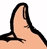There is considerable overlap between waveguide standards, you can almost always find two types that will work at one frequency. In order to get the lowest loss, choose the waveguide that has the largest dimensions.

Loss due to dielectric loss tangent

Ordinarily waveguides are filled only with air, so the loss tangent loss is essentially zero. But once in a while you'll find a reaon to consider a dielectric-filled waveguide, then the properties of the dielectric must be considered. This topic is covered on a separate page.

Author : Unknown Editor